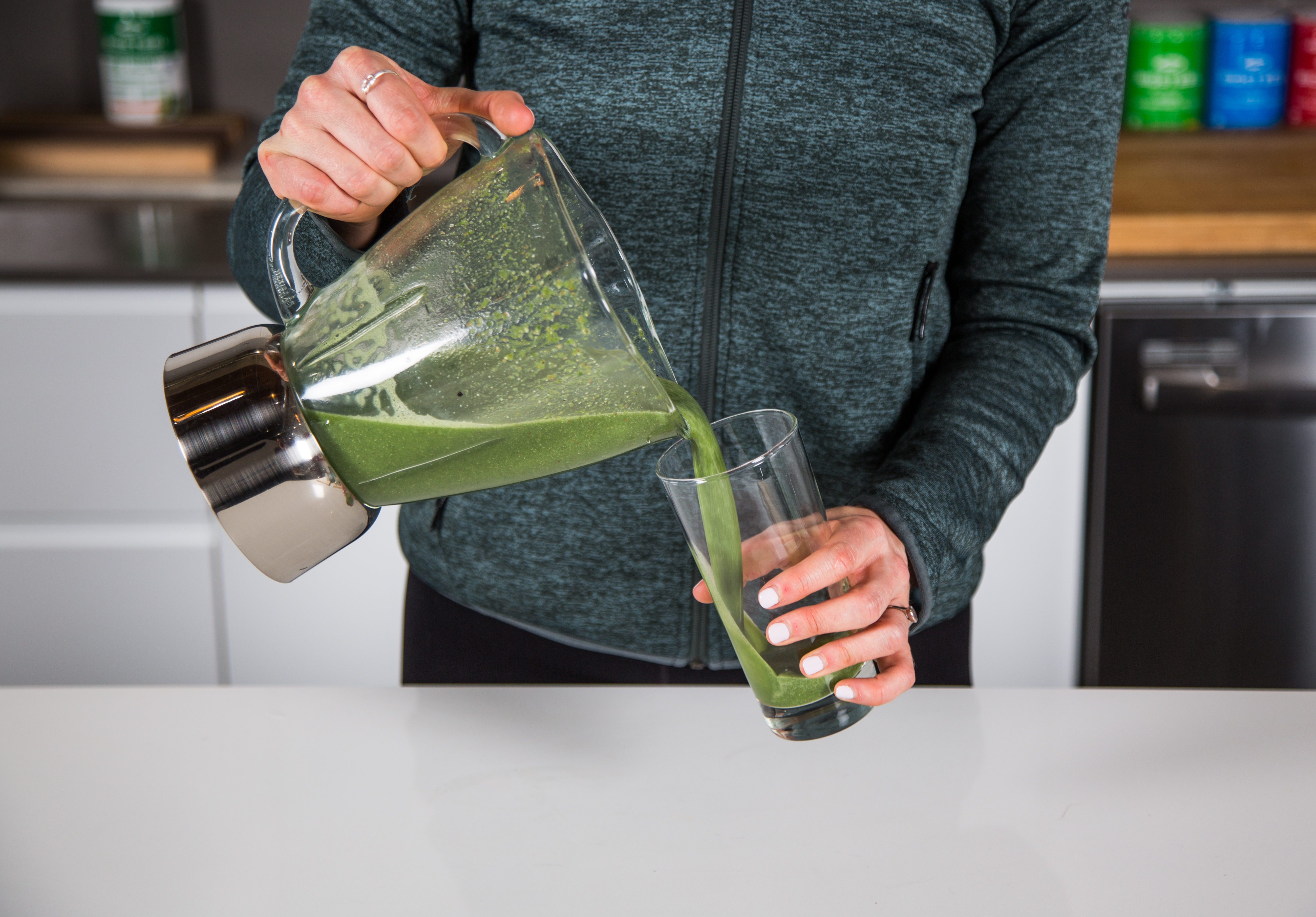# Eugenie Bouchard

Here is a caption.

Here is a caption.

Here is a caption.

Here is a caption.

Here is a caption. Here is a caption. Here is a caption. Here is a caption. Here is a caption. Here is a caption. Here is a caption. Here is a caption. Here is a caption. Here is a caption. Here is a caption. Here is a caption. Here is a caption. Here is a caption. Here is a caption. Here is a caption. Here is a caption. Here is a caption. Here is a caption. Here is a caption. Here is a caption. Here is a caption. Here is a caption. Here is a caption. Here is a caption.

CAPTION THIS.